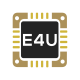# Types of Control Systems | Linear and Non Linear Control System

A control system is a system of devices that manages, commands, directs or regulates the behavior of other devices to achieve a desired result. In other words, the definition of a control system can be simplified as a system which controls other systems to achieve a desired state. There are various types of control systems, which can be broadly categorised as linear control systems or non-linear control systems. These types of control systems are discussed in detail below.

### Linear Control Systems

In order to understand the linear control system, we should first understand the principle of superposition. The principle of superposition theorem includes two the important properties and they are explained below:
Homogeneity: A system is said to be homogeneous, if we multiply input with some constant A then the output will also be multiplied by the same value of constant (i.e. A).
Additivity: Suppose we have a system S and we are giving the input to this system as a1 for the first time and we are getting the output as b1 corresponding to input a1. On the second time we are giving input a2 and correspond to this we are getting the output as b2.

Now suppose this time we are giving input as a summation of the previous inputs (i.e. a1 + a2) and corresponding to this input suppose we are getting the output as (b1 + b2) then we can say that system S is following the property of additivity. Now we are able to define the linear control systems as those types of control systems which follow the principle of homogeneity and additivity.

#### Examples of Linear Control System

Consider a purely resistive network with a constant DC source. This circuit follows the principle of homogeneity and additivity. All the undesired effects are neglected and assuming ideal behavior of each element in the network, we say that we will get linear voltage and current characteristic. This is the example of a linear control system.

### Non-linear Control Systems

We can simply define a nonlinear control system as a control system which does not follow the principle of homogeneity. In real life, all control systems are non-linear systems (linear control systems only exist in theory). The describing function is an approximate procedure for analyzing certain nonlinear control problems.

#### Examples of Non-linear System

A well-known example of a non-linear system is a magnetization curve or no load curve of a DC machine. We will discuss briefly no-load curve of DC machines here: No load curve gives us the relationship between the air gap flux and the field winding mmf. It is very clear from the curve given below that in the beginning, there is a linear relationship between winding mmf and the air gap flux but after this, saturation has come which shows the nonlinear behavior of the curve or characteristics of the nonlinear control system.### Analog or Continuous System

In these types of control systems, we have a continuous signal as the input to the system. These signals are the continuous function of time. We may have various sources of continuous input signal like sinusoidal type signal input source, square type of signal input source; the signal may be in the form of continuous triangle etc.

### Digital or Discrete System

In these types of control systems, we have a discrete signal (or signal may be in the form of pulse) as the input to the system. These signals have a discrete interval of time. We can convert various sources of continuous input signal like sinusoidal type signal input source, square type of signal input source etc into a discrete form using the switch.
Now there are various advantages of discrete or digital system over the analog system and these advantages are written below:

1. Digital systems can handle nonlinear control systems more effectively than the analog type of systems.
2. Power requirement in case of a discrete or digital system is less as compared to analog systems.
3. Digital system has a higher rate of accuracy and can perform various complex computations easily as compared to analog systems.
4. Reliability of the digital system is more as compared to an analog system. They also have a small and compact size.
5. Digital system works on the logical operations which increases their accuracy many times.
6. Losses in case of discrete systems are less as compared to analog systems in general.

### Single Input Single Output Systems

These are also known as SISO type of system. In this, the system has single input for a single output. Various example of this kind of system may include temperature control, position control system, etc.

### Multiple Input Multiple Output Systems

These are also known as MIMO type of system. In this, the system has multiple outputs for multiple inputs. Various example of this kind of system may include PLC type system etc.

### Lumped Parameter System

In these types of control systems, the various active and passive components are assumed to be concentrated at a point and that’s why these are called lumped parameter type of system. Analysis of such type of system is very easy which includes differential equations.

### Distributed Parameter System

In these types of control systems, the various active (like inductors and capacitors) and passive parameters (resistor) are assumed to be distributed uniformly along the length and that’s why these are called distributed parameter type of system. Analysis of such type of system is slightly difficult which includes partial differential equations.

Want To Learn Faster? 🎓
Get electrical articles delivered to your inbox every week.
No credit card required—it’s 100% free.